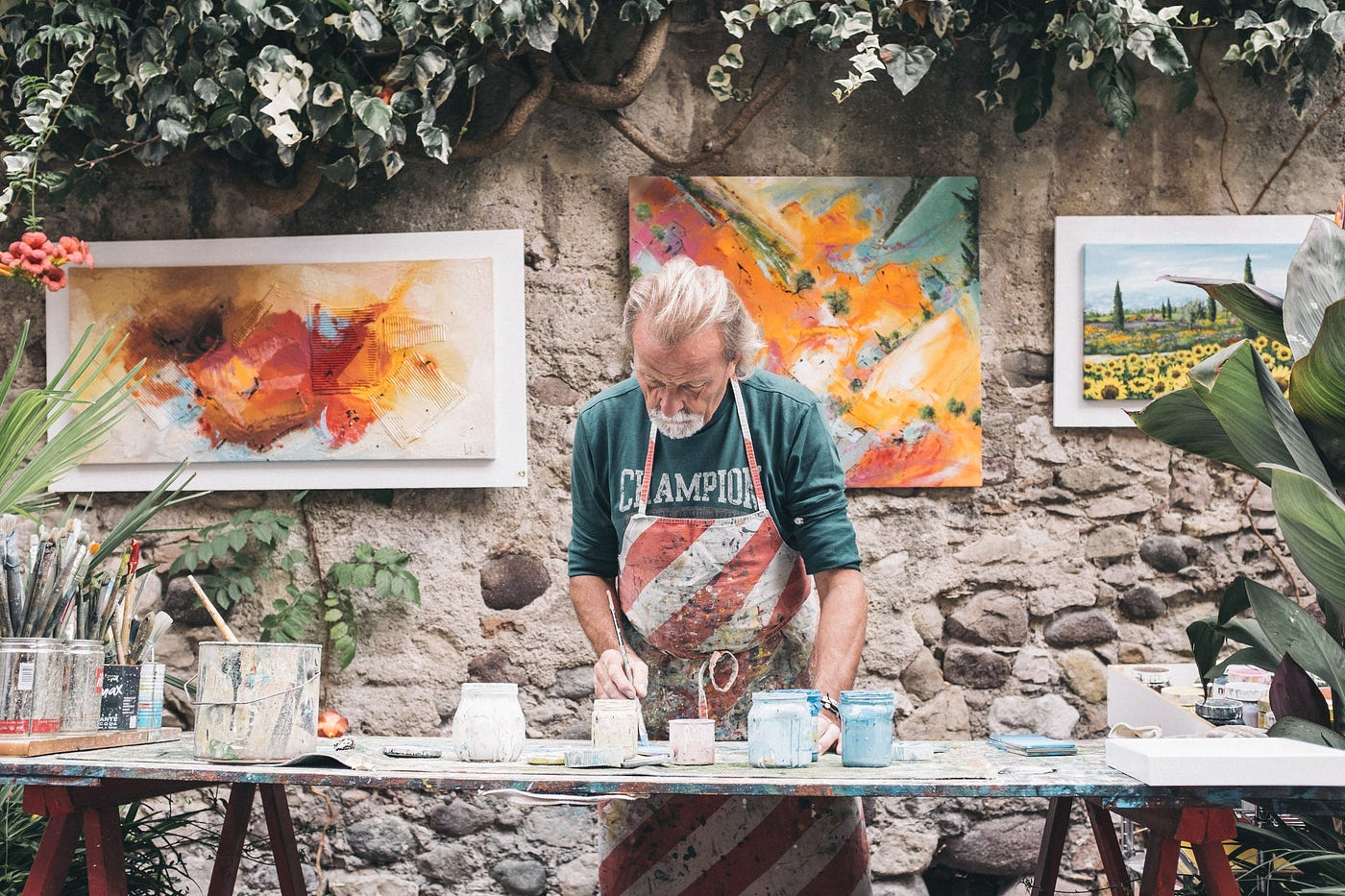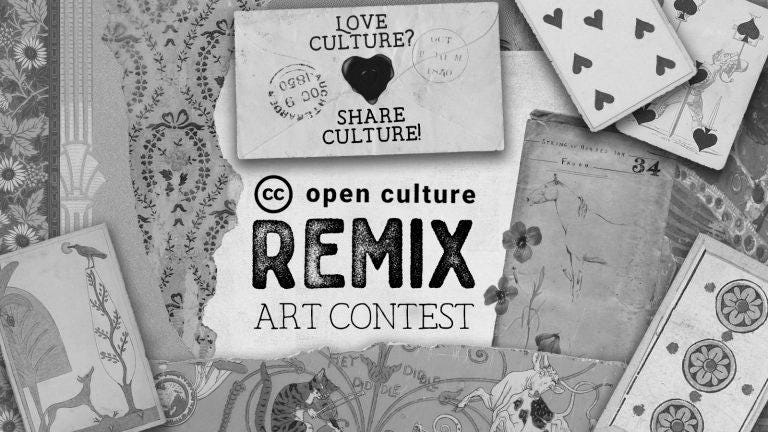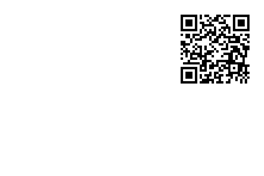# Golang: Creating Images and Drawing — Simply Explained (Part 1)Photo by Eddy Klaus on Unsplash

# Understanding the key elements of the framework

Golang is useful and oft mentioned for it’s web development functions. So let’s take sometime to understand the components of the imaging framework to get the lay of the land.

## Interface image.Image

The interface is what you need to know first. Any types in Golang can be said to follow the image.Image interface if it implements:

1. Function that returns a color model — this color model is how the data translating to pixels are stored in binary format;
2. Function that returns the bounding rectangle — this represents the size of the rectangle that forms the bounding geometry of the image; and
3. Function that returns the color.Color at the input x and y integer coordinates.
`type Image interface {		ColorModel() color.Model		Bounds() Rectangle		At(x, y int) color.Color}`

## Interface draw.Image

The image.Image interface with the At function is like a read only image. It can only tell you the color at each coordinate of the pixel. What if we want to create an image. That’s where the interface draw.Image comes in under the image/draw package.

`type Image interface {	image.Image	Set(x, y int, c color.Color)}`

## Actual Structs

The structs that support the two aforementioned interfaces are listed below:

• Gray —8-bit greyscale
• Gray16– 16–bit greyscale
• CMYK
• Paletted
• RGBA — most commonly know color model of red, green, blue and alpha channels, each 8 bits
• NRGBA — non-alpha-premultiplied 32-bit color (each channel is 8-bit)
• NRGBA64 — non-alpha-premultiplied 64-bit color (each channel is 16-bit)
• NYCbCrA — non-alpha-premultiplied Y’CbCr-with-alpha (8-bit for each of Luma and the two chroma components; JPEG, VP8, MPEG codecs etc use this color model)
• Alpha — a single channel (alpha) 8-bit
• Alpha16 — a single channel (alpha) 16-bit

## Now that I know about the structs, but how do you get the structs then?

We have to look at packages such as image/jpeg and image/png etc. These are packages that allow you to open say a jpeg image file and Decode the file into image.Image instances. Example code below:

`import _ "image/jpeg" //will call the init() function of the package                      //thus enabling working with jpeg filefunc OpenImage(path string) (image.Image, error) {        f, err := os.Open(path)        if err != nil {                fmt.Println(err)                return nil, err        }        defer f.Close()img, format, err := image.Decode(f)        if err != nil {                e := fmt.Errorf("error in decoding: %w", err)                return nil, e        }if format != "jpeg" && format != "png" {                e := fmt.Errorf("error in image format - not jpeg")                return nil, e        }return img, nil}`
`func GetImageTensor(img image.Image) (pixels [][]color.Color) {        size := img.Bounds().Size()        for i := 0; i < size.X; i++ {                var y []color.Color                for j := 0; j < size.Y; j++ {                        y = append(y, img.At(i, j))                }                pixels = append(pixels, y) // 2 by 2 slices where                                       //each contains a color.color        }        return}`
`func ConvertGreyScale(pixels *[][]color.Color) image.Image {        p := *pixels        wg := sync.WaitGroup{}        rect := image.Rect(0, 0, len(p), len(p))        newImage := image.NewRGBA(rect)        for x := 0; x < len(p); x++ {                for y := 0; y < len(p); y++ {                        wg.Add(1)                        go func(x, y int) {                                pix := p[x][y]                                originalColor, ok := color.RGBAModel.Convert(pix).(color.RGBA)                                if !ok {                                        log.Fatalf("color.color conversion went wrong")                                }                                grey := uint8(float64(originalColor.R)*0.21 + float64(originalColor.G)*0.72 + float64(originalColor.B)*0.07)                                col := color.RGBA{                                        grey,                                        grey,                                        grey,                                        originalColor.A,                                }                                newImage.Set(x, y, col)        wg.Done()                        }(x, y)                }        }        return newImage}`Original image (courtesy of CC) and the grayscaled imageExample of the draw library where you can place your own QR code through compositing

--

--